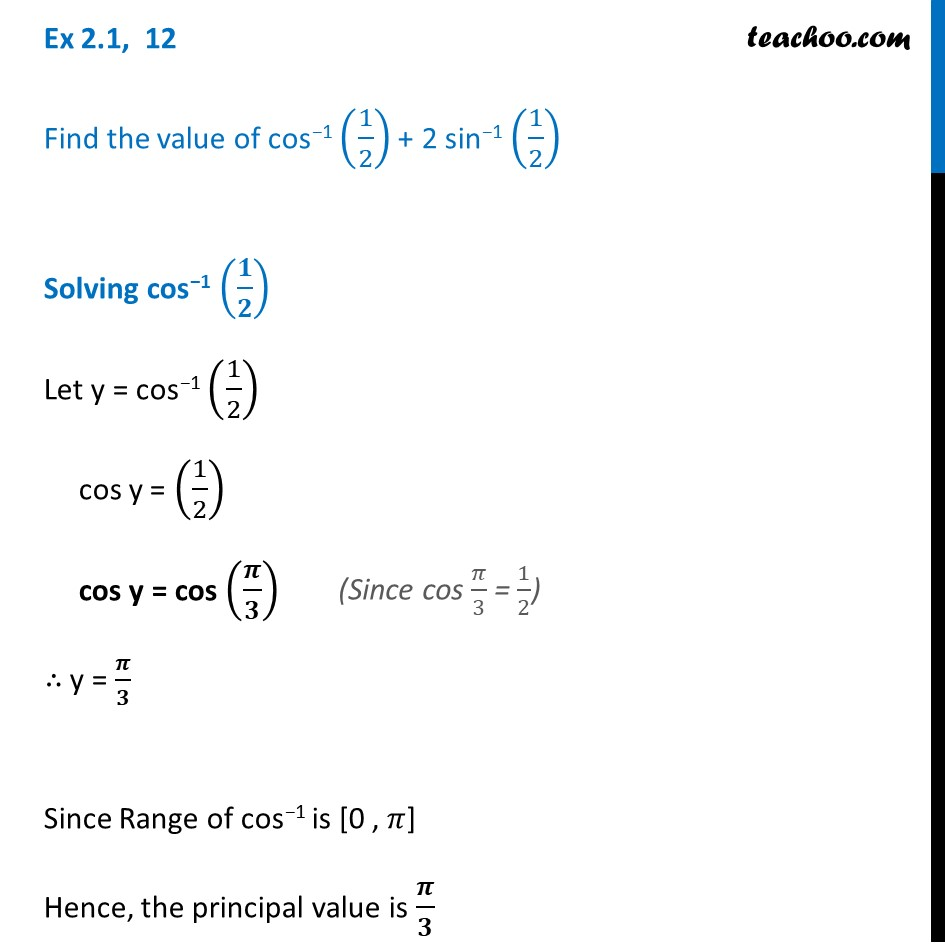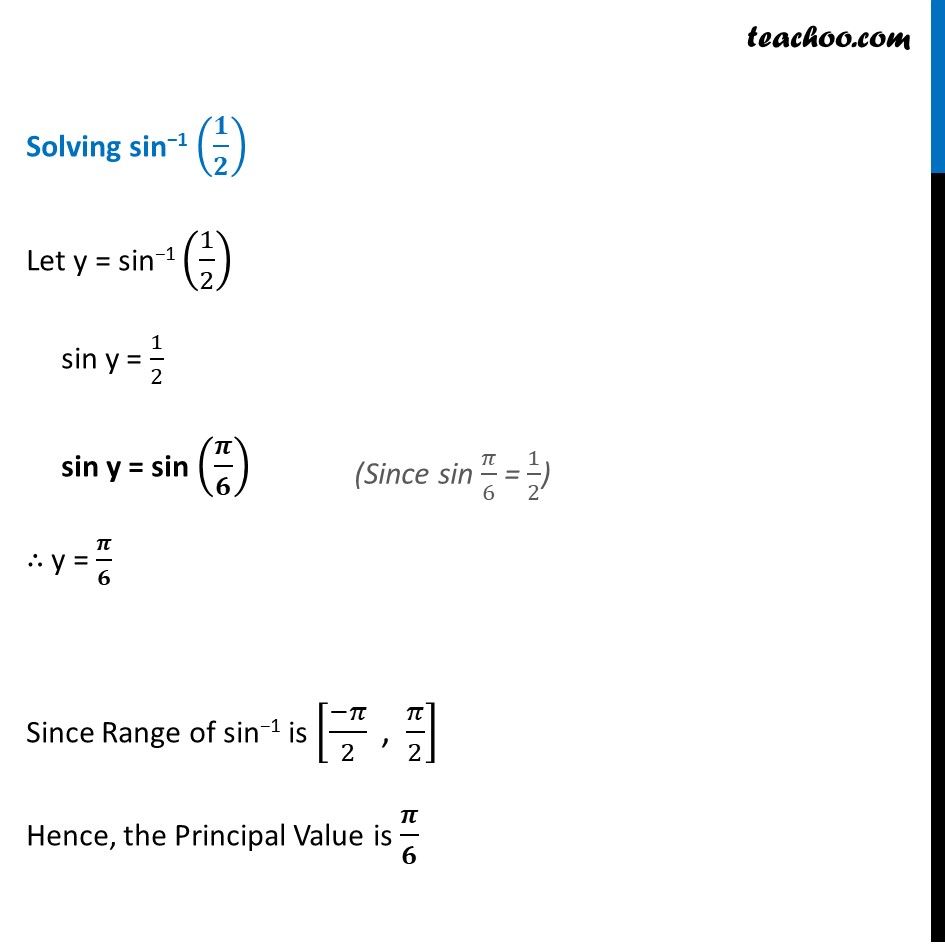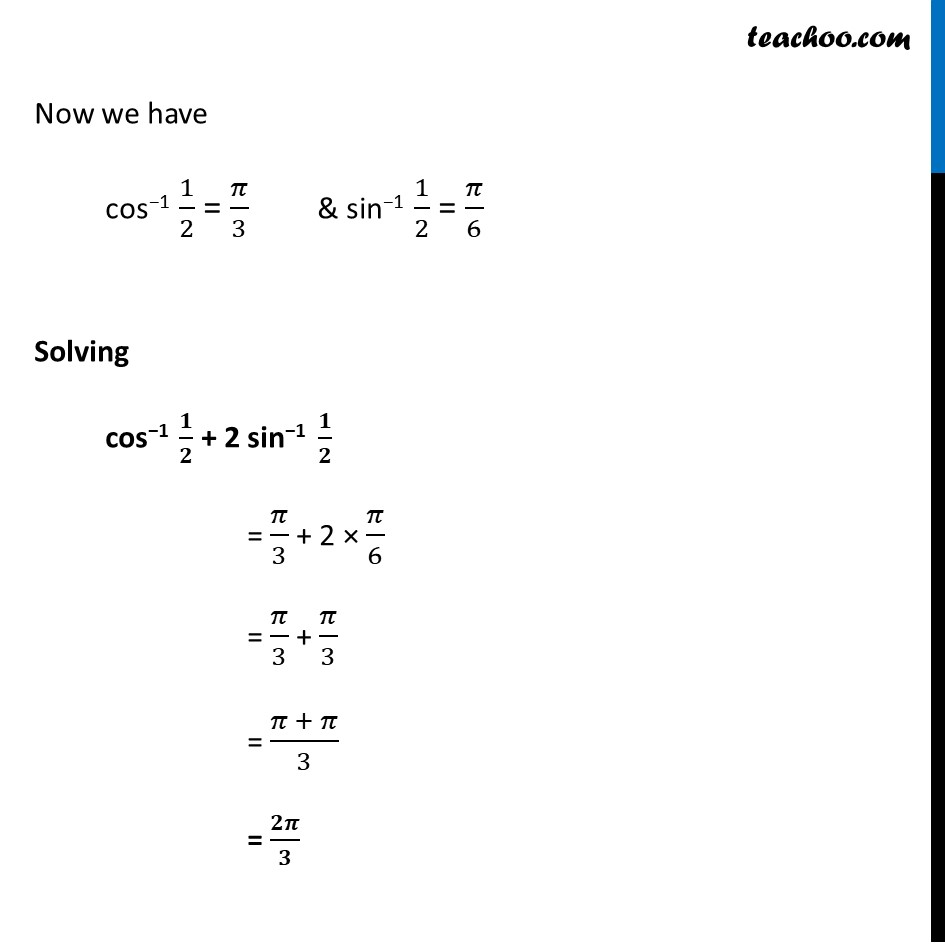Chapter 2 Class 12 Inverse Trigonometric Functions

Class 12
Important Questions for exams Class 12Learn in your speed, with individual attention - Teachoo Maths 1-on-1 Class

### Transcript

Ex 2.1, 12 Find the value of cos−1 (1/2) + 2 sin−1 (1/2) Solving cos−1 (𝟏/𝟐) Let y = cos−1 (1/2) cos y = (1/2) cos y = cos (𝝅/𝟑) ∴ y = 𝝅/𝟑 Since Range of cos−1 is [0 , 𝜋] Hence, the principal value is 𝝅/𝟑 (Since cos 𝜋/3 = 1/2) Solving sin−1 (𝟏/𝟐) Let y = sin−1 (1/2) sin y = 1/2 sin y = sin (𝝅/𝟔) ∴ y = 𝝅/𝟔 Since Range of sin−1 is [(−𝜋)/2 " , " 𝜋/2] Hence, the Principal Value is 𝝅/𝟔 (Since sin 𝜋/6 = 1/2) Now we have cos−1 1/2 = 𝜋/3 & sin−1 1/2 = 𝜋/6 Solving cos−1 𝟏/𝟐 + 2 sin−1 𝟏/𝟐 = 𝜋/3 + 2 × 𝜋/6 = 𝜋/3 + 𝜋/3 = (𝜋 + 𝜋)/3 = 𝟐𝝅/𝟑